## Example Questions

### Example Question #1 : How To Find The Angle For A Percentage Of A Circle

An ant begins at the center of a pie with a 12" radius. Walking out to the edge of pie, it then proceeds along the outer edge for a certain distance. At a certain point, it turns back toward the center of the pie and returns to the center point. Its whole trek was 55.3 inches.  What is the approximate size of the angle through which it traveled?

81.53°

149.52°

128.21°

74.76°

91.44°

149.52°

Explanation:

To solve this, we must ascertain the following:

1) The arc length through which the ant traveled.

2) The percentage of the total circumference in light of that arc length.

3) The percentage of 360° proportionate to that arc percentage.

To begin, let's note that the ant travelled 12 + 12 + x inches, where x is the outer arc distance. (It traveled the radius twice, remember); therefore, we know that 24 + x = 55.3 or x = 31.3.

Now, the total circumference of the circle is 2πr or 24π.  The arc is 31.3/24π percent of the total circumference; therefore, the percentage of the angle is 360 * 31.3/24π. Since the answers are approximations, use 3.14 for π. This would be 149.52°.

### Example Question #11 : Sectors

A study was conducted to determine the effectiveness of a vaccine for the common cold (Rhinovirus sp.). 1000 patients were studied. Of those, 500 received the vaccine and 500 did not.  The patients were then exposed to the Rhinovirus and the results were tabulated.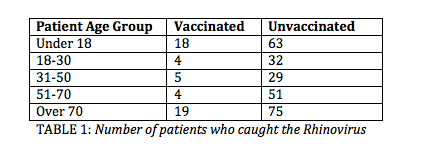Table 1 shows the number of vaccinated and unvaccinated patients in each age group who caught the cold.

Suppose the scientists wish to create a pie chart reflecting a patient's odds of catching the virus depending on vaccination status and age group.

All 1000 patients are included in this pie chart.

What would be the angle of the arc for the portion of the chart representing vaccinated patients of all age groups who caught the virus?

18°

36°

Insufficient information to answer this question

60°

10°

18°

Explanation:

First, we must determine what proportion of the 1000 patients were vaccinated and caught the virus.  The total number of patients who were vaccinated and caught the virus is 50.

18 + 4 + 5 + 4 + 19 = 50

The proportion of the patients is represented by dividing this group by the total number of participants in the study.

50/1000 = 0.05

Next, we need to figure out how that proportion translates into a proportion of a pie chart.  There are 360° in a pie chart.  Multiply 360° by our proportion to reach the solution.

360° * 0.05 = 18°

The angle of the arc representing vaccinated patients who caught the virus is 18°.

### Example Question #12 : Sectors

A group of students ate an-inch pizza that was cut intoequal slices. What was the angle measure needed to cut this pizza into these equal slices?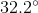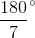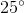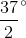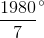You will not need all of the information given in the prompt in order to answer this question successfully. You really only need to know that there wereslices. If the slices were evenly divided among the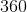degrees of the pizza, this means that the degree measure of each slice was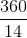. This reduces to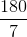degrees.